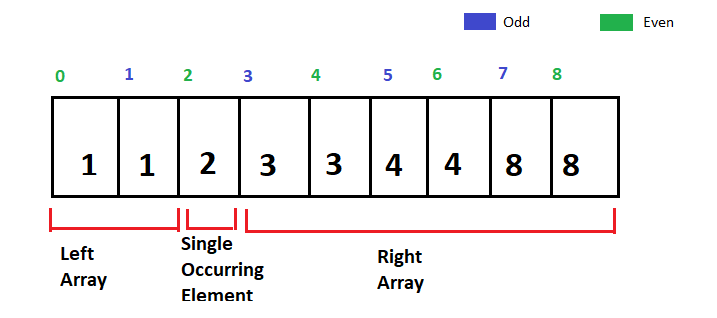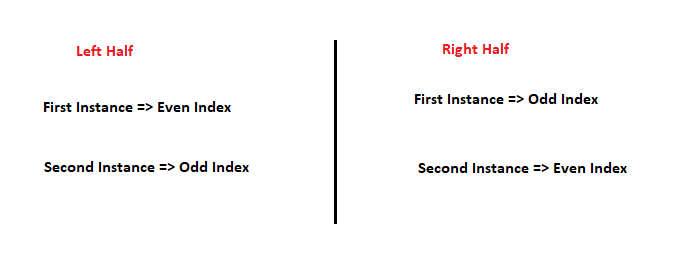# Search Single Element in a sorted array

Problem Statement: Given a sorted array of N integers, where every element except one appears exactly twice and one element appears only once. Search Single Element in a sorted array.

Example 1:

```Input: N = 9, array[] = {1,1,2,3,3,4,4,8,8}

Output: 2

Explanation: Every element in this sorted array except 2
appears twice, therefore the answer returned will be 2.```

Example 2:

```Input: N = 7, array[] = {11,22,22,34,34,57,57}

Output: 11

Explanation: Every element in this sorted array except
11 appears twice, therefore the answer returned will be 11.```

Solution

Disclaimer: Don’t jump directly to the solution, try it out yourself first.

Solution 1: Using XOR(^)

Approach: As every number in the array repeats twice and only one number occurs once, we can take advantage of the XOR(^) operator. These are two properties of the XOR operator which will be helpful.

If p is a number then,

p^p=0

p^0=p

If we find the XOR of every element of the array, we will get the answer.

Code:

## C++ Code

``````#include<bits/stdc++.h>

using namespace std;
class Solution {
public:
int findSingleElement(vector < int > & nums) {

int n = nums.size();
int elem = 0;
for (int i = 0; i < n; i++) {
elem = elem ^ nums[i];
}

return elem;
}
};

int main() {
Solution obj;
vector < int > v {1,1,2,3,3,4,4,8,8};

int elem = obj.findSingleElement(v);

cout << "The single occurring element is "
<< elem << endl;
}``````

Output:

The single occurring element is 2

Time Complexity: O(N)

Space Complexity: O(1)

## Java Code

``````import java.util.*;
class TUF {
public static int findSingleElement(int nums[]) {
int n = nums.length;
int elem = 0;
for (int i = 0; i < n; i++) {
elem = elem ^ nums[i];
}

return elem;
}

public static void main(String args[]) {

int arr[] = {1,1,2,3,3,4,4,8,8};

int elem = findSingleElement(arr);
System.out.println("The single occurring"
+" element is " + elem);

}
}``````

Output:

The single occurring element is 2

Time Complexity: O(N)

Space Complexity: O(1)

Solution 2:

Approach: Using Binary Search

As the elements are sorted, twice occurring elements will be placed together in the input array. Moreover, the input array has one element occurring once, therefore a general input can be thought of like this.Now in this left array, the first instance of every element is occurring on the even index and the second instance on the odd index. Similarly in the right array, the first instance of every element is occurring on the odd index and the second index is occurring on the even index. This is summarized below.So the algorithmic approach will be to use binary search. The intuition is that when we see an element, if we know its index and whether it is the first instance or the second instance, we can decide whether we are presently in the left array or right array. Moreover, we can decide which direction we need to move to find the breakpoint. We need to find this breakpoint between our left array and the right array.

We will check our mid element, if it is in the left array, we will shrink our left array to the right of this mid element, if it is in the right array, we will shrink the right array to the left of this mid element. This binary search process will continue till the right array surpasses our left one and the low is pointing towards the breakpoint.

Dry Run:   In case you want to see the dry run of this approach, please watch the video at the bottom.

Code:

## C++ Code

``````#include<bits/stdc++.h>

using namespace std;
class Solution {
public:
int findSingleElement(vector < int > & nums)
{
int low = 0;
int high = n - 2;

while (low <= high) {
int mid = (low + high) / 2;

if (mid % 2 == 0) {
if (nums[mid] != nums[mid + 1])
//Checking whether we are in right half

high = mid - 1; //Shrinking the right half
else
low = mid + 1; //Shrinking the left half
} else {

//Checking whether we are in right half
if (nums[mid] == nums[mid + 1])
high = mid - 1; //Shrinking the right half
else
low = mid + 1; //Shrinking the left half
}
}

return nums[low];
}
};

int main() {
Solution obj;
vector < int > v {1,1,2,3,3,4,4,8,8
};

int elem = obj.findSingleElement(v);
cout << "The single occurring element is " +
" << elem << endl;

}``````

Output:

The single occurring element is 2

Time Complexity: O(log(N))

Space Complexity: O(1)

## Java Code

``````import java.util.*;
class TUF {
static int findSingleElement(int nums[]) {

int low = 0;
int high = nums.length - 2;

while (low <= high) {
int mid = (low + high) / 2;
if (mid % 2 == 0) {
// Checking whether we are in right half
if (nums[mid] != nums[mid + 1])

//Shrinking the right half
high = mid - 1;
else
// Shrinking the left half
low = mid + 1;
} else {
// Checking whether we are in right half
if (nums[mid] == nums[mid + 1])
//Shrinking the right half
high = mid - 1;
else
// Shrinking the left half
low = mid + 1;
}
}
return nums[low];
}

public static void main(String args[]) {

int arr[] = {1,1,2,3,3,4,4,8,8};

int elem = findSingleElement(arr);

System.out.println("The single occurring" +
" element is " + elem);

}
}``````

Output:

The single occurring element is 2

Time Complexity: O(log(N))

Space Complexity: O(1)